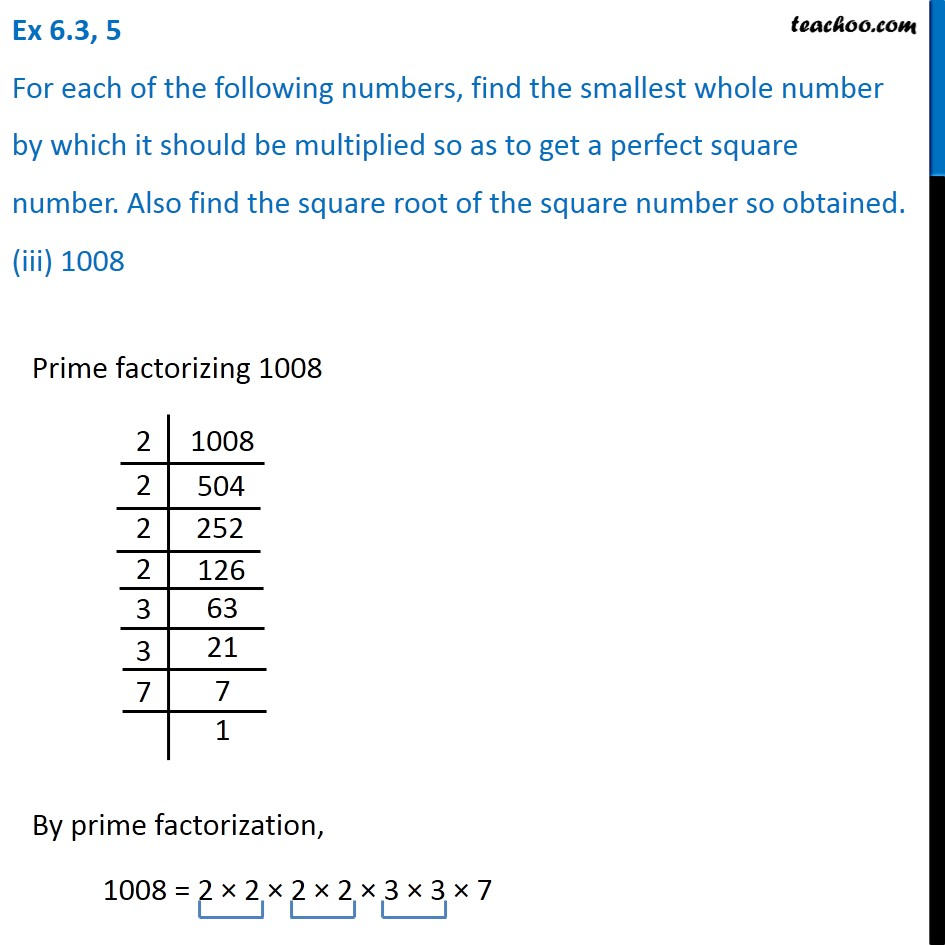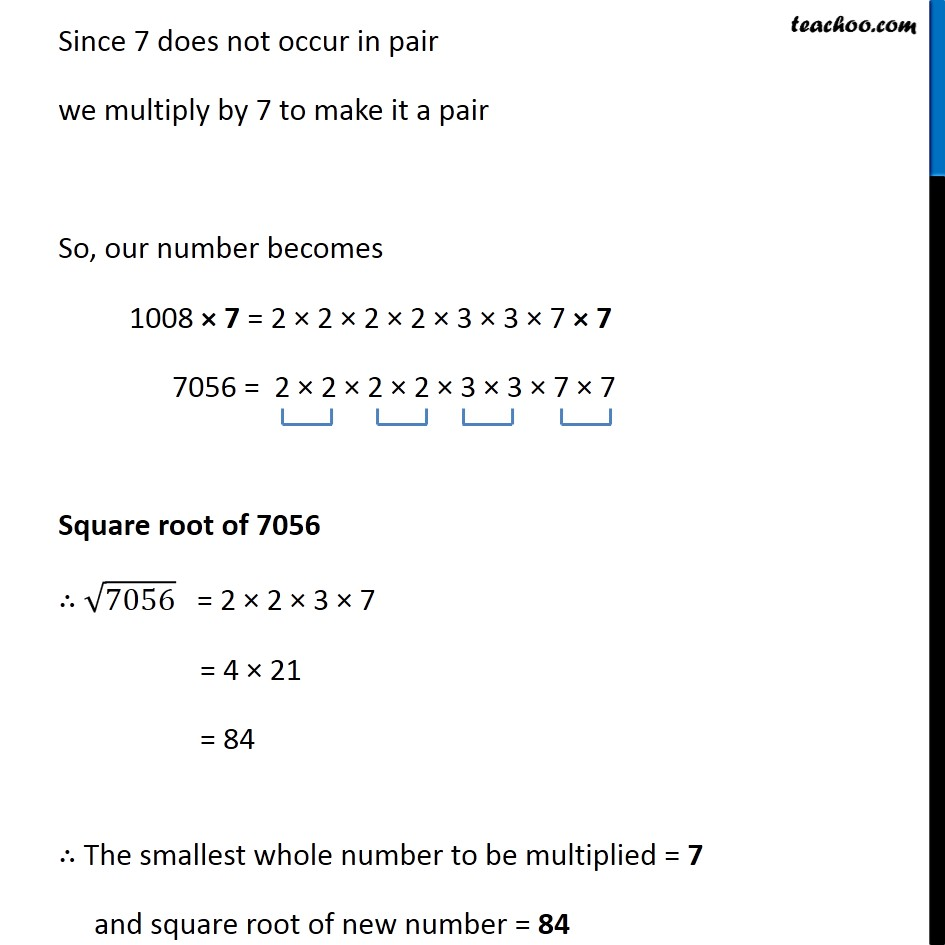Ex 6.3

Chapter 6 Class 8 Squares and Square Roots
Serial order wise### Transcript

Ex 6.3, 5 For each of the following numbers, find the smallest whole number by which it should be multiplied so as to get a perfect square number. Also find the square root of the square number so obtained. (iii) 1008 Prime factorizing 1008 By prime factorization, 1008 = 2 × 2 × 2 × 2 × 3 × 3 × 7 Since 7 does not occur in pair we multiply by 7 to make it a pair So, our number becomes 1008 × 7 = 2 × 2 × 2 × 2 × 3 × 3 × 7 × 7 7056 = 2 × 2 × 2 × 2 × 3 × 3 × 7 × 7 Square root of 7056 ∴ √7056 = 2 × 2 × 3 × 7 = 4 × 21 = 84 ∴ The smallest whole number to be multiplied = 7 and square root of new number = 84